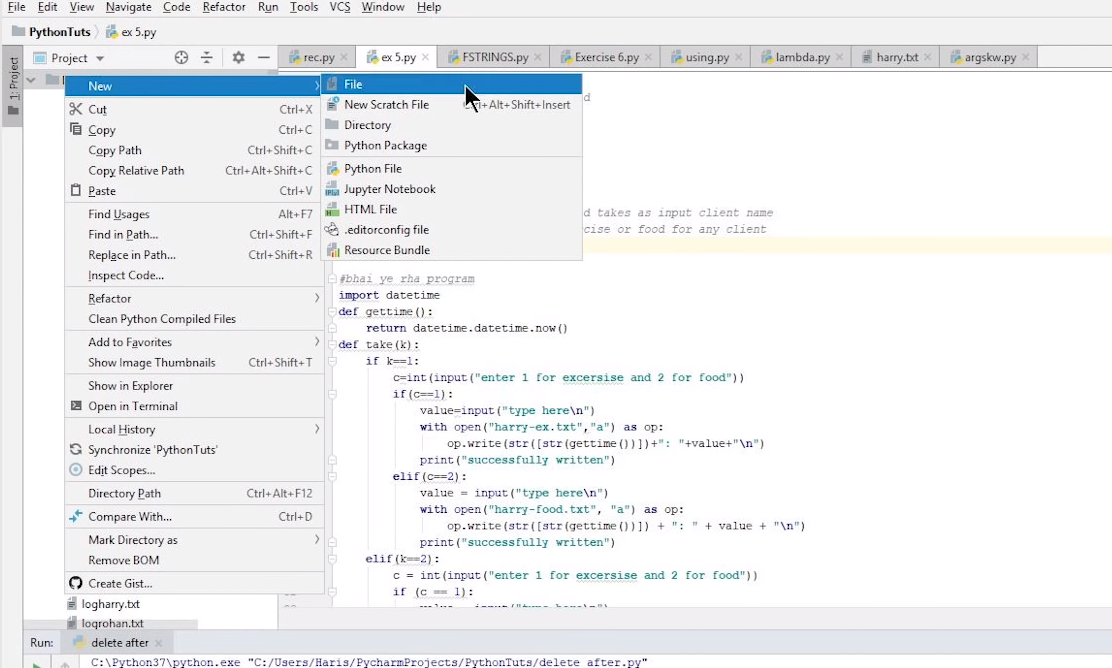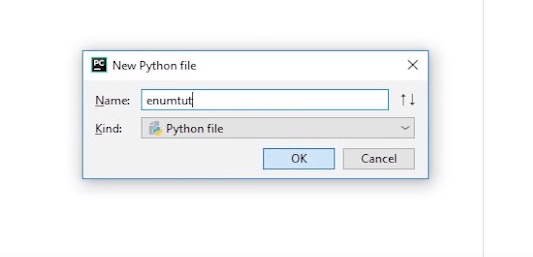### Enumerate Function

So guys, you must already have noticed that we are using Pycharm in all of our python tutorials. So it will be best if you use the same ide, so you can keep up with me in every way.Note: Do not name your file similar to a module name. The reason is explained un Tutorial #45. Give it a read or watch the video for further clarification.We are going to name our file enumtut here which means enumerate tutorial. So, let's get started without wasting time.

“ An enumerate is a built-in function that provides an index to data structure elements, making iterations through them easier and simpler.”

In previous tutorials, we have seen many methods that print items of different data structures onto the screen. For these kinds of operations, we need to have a for loop along with an iterator and an integer variable in which we have to increment every time the loop runs. Well, python provides us with a simple and easy solution to deal with certain situations by providing us with a built-in function known as “enumerate function.” Using the enumerate function, we can summarize the code and make it easier and simpler to use. It is really important to know the concept of Enumerate function so that you can apply this concept to your code.

#### Let us understand the working of enumerate function:

What enumerate function does is, it assigns an index to every element or value in the object that we want to iterate, so we do not have to assign a specific variable for incremental function, instead we have to apply a for loop, and our function will start working. Its syntax is a lot simpler and shorter than what we have been following till now.

#### Syntax

``enumerate(iterable, start=0)``

When calling a simple enumeration function, we have to provide two parameters:

• The data structure that we want to iterate
• The index from where we want to start our iteration

Note: The iterable must be an object that supports iteration

#### Example of enumerate using a python list.

We can iterate over the index and value of an item in a list by using a basic for loop with enumerate().

``````list_1=["code","with","harry"]
for index,val in enumerate(list_1):
print(index,val)``````

#### Output:

``````0 code
1 with
2 harry``````

#### Using Enumerate() on a list with start Index:

In the below example, the starting index is given as 5. The index of the first item will start from the given starting index.

#### Example:

``````list_2 = ["Python", "Programming", "Is", "Fun"]
#Counter value starts from 5
result = enumerate(list_2, 5)
print(list(result))``````

We will get the following output:

``[(5, 'Python'), (6, 'Programming'), (7, 'Is'), (8, 'Fun')]``

If we do not provide the index, we want to start the iteration from then it automatically starts its iteration from zero index i.e., the beginning of the data structure.

Instead of returning a string, the enumerate function returns an object by adding the iterating counter value. We can also convert the enumerator object into a list(), tuple(), set(), and many more.

• It is a built-in function
• It makes the code shorter
• We do not have to keep count of the number of iterations
• It makes the implementation of for loop simpler and cleaner
• Lesser code so lessor chances of error and bugs
• We can loop through string, tuple or objects using enumerate
• We can start the iteration from anywhere within the data structure as we have the option of providing the starting index for iteration.

#### Code file as described in the video

``````l1 = ["Bhindi", "Aloo", "chopsticks", "chowmein"]

# i = 1
# for item in l1:
#     if i%2 is not 0:
#     i += 1

for index, item in enumerate(l1):
if index%2==0:

``````